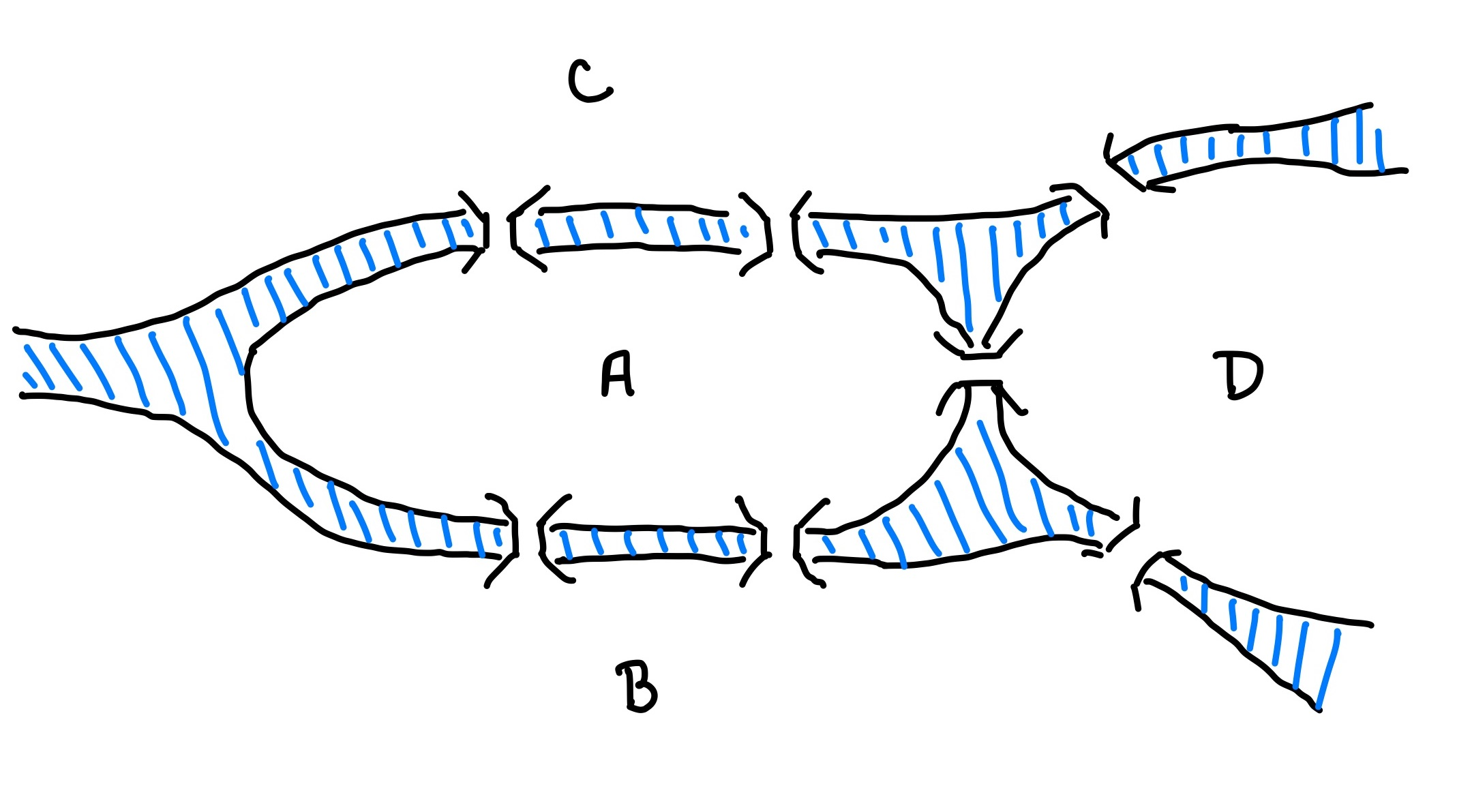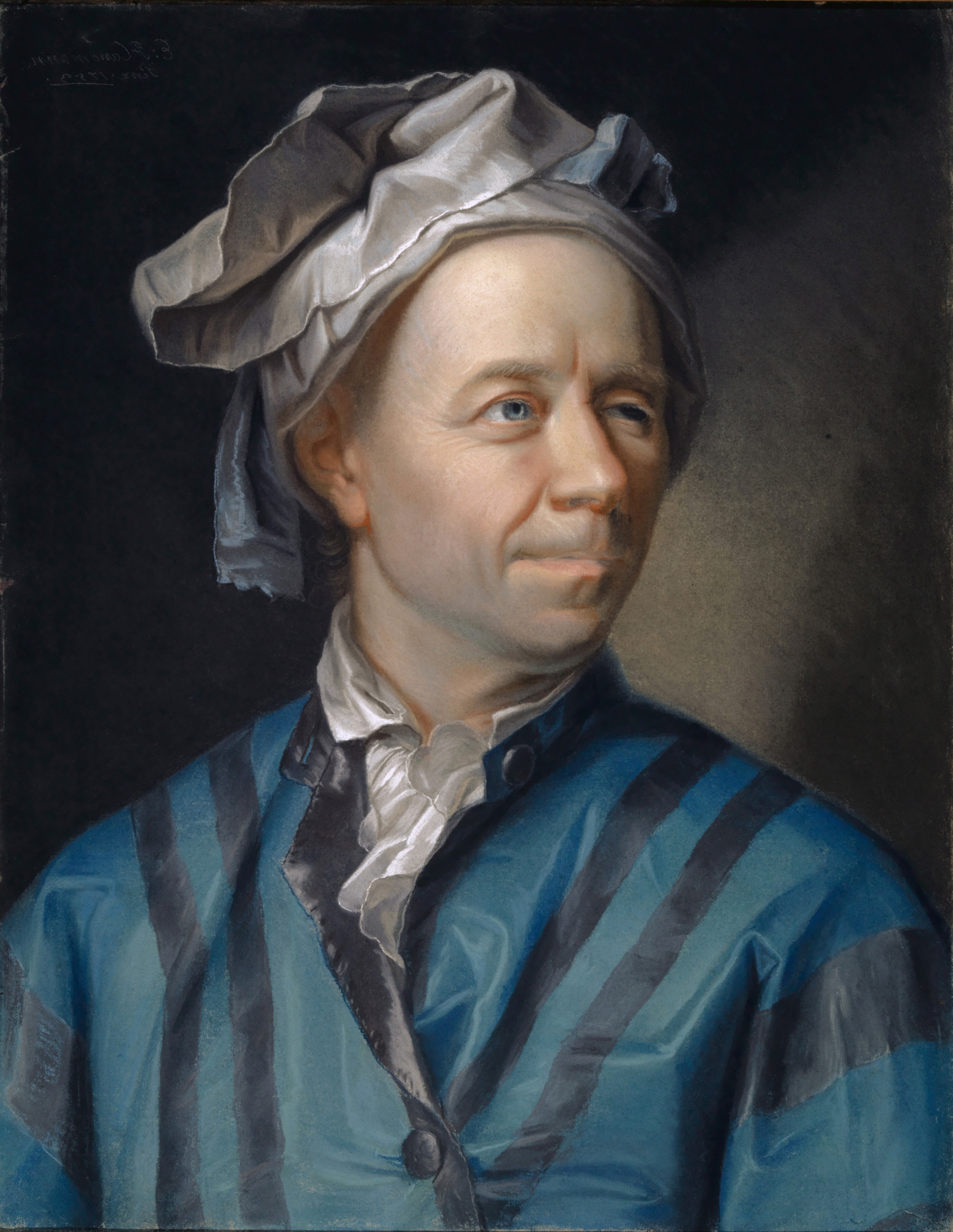# Lecture 13: Bridges, Graphs, and Greed

### COSC 311 Algorithms, Fall 2022

$\def\compare{ {\mathrm{compare}} } \def\swap{ {\mathrm{swap}} } \def\sort{ {\mathrm{sort}} } \def\insert{ {\mathrm{insert}} } \def\true{ {\mathrm{true}} } \def\false{ {\mathrm{false}} } \def\BubbleSort{ {\mathrm{BubbleSort}} } \def\SelectionSort{ {\mathrm{SelectionSort}} } \def\Merge{ {\mathrm{Merge}} } \def\MergeSort{ {\mathrm{MergeSort}} } \def\QuickSort{ {\mathrm{QuickSort}} } \def\Split{ {\mathrm{Split}} } \def\Multiply{ {\mathrm{Multiply}} } \def\Add{ {\mathrm{Add}} } \def\cur{ {\mathrm{cur}} }$

## Announcements

1. Midterm next Friday
• Material up to lecture 12 covered
• Makeup date following week
• Accommodations
2. Study guide posted this weekend
• Topics
• Example questions
• Solutions

## Overview

1. Bridges of Königsberg
2. Graphs
3. Greedy Algorithms

## Bridges of KönigsbergQuestion. Is it possible to walk around Königsberg, cross every bridge exactly once, and return to where you started?

## A Result

Question. Is it possible to walk around Königsberg, cross every bridge exactly once, and return to where you started?Theorem (Euler, 1736). No.

## Abstraction

1. Replace each separate landmass with a single point
• all points in each landmass are reachable from each other without crossing a bridge
2. Represent each bridge with an “edge” connecting landmasses## Graphs

A graph $G = (V, E)$ consists of

1. a set $V$ of vertices or nodes
2. a set $E$ of edges, where each edge consists of a pair of nodes
• if $e = (u, v)$ an edge, we say $u$ and $v$ are adjacent
• $G$ a multigraph if multiple edges between same pair of vertices

Example. Königsberg graph

## Paths and Circuits

Note. Terminology varies from source to source.

A path $P$ of length $k$ in $G$ is a sequence of the form $v_0 e_1 v_1 e_2 v_2 \cdots e_k v_k$ where

• each $v_i$ is a vertex, and
• each $e_i$ is an edge with $e_i = (v_{i-1}, v_i)$.

$G$ is connected if for every pair of vertices $u, v \in V$, there is a path from $u$ to $v$.

$P$ is a circuit if $v_0 = v_k$.

## BoK as a Graph Problem

Original Question. Is it possible to walk around Königsberg, cross every bridge exactly once, and return to where you started?

Rephrasing as Graph Problem. Given a graph $G = (V, E)$, is there a circuit that contains every edge $e \in E$ exactly once?

• A graph with this property is called Eulerian.

## General Questions

Question 1. Under what conditions is a graph $G$ Eulerian?

Question 2. If $G$ is Eulerian, how can we find an Eulerian circuit?

## Degrees

Let $G = (V, E)$ be a graph, and $v \in V$ a vertex. The degree of $v$, $\deg(v)$ is the number of edges in $E$ incident to $v$.

• $G$ is even if all vertices have even degree

## A Necessary Condition

Claim (Euler 1736). If $G$ is Eulerian, then every vertex has even degree.

Why?

• Suppose $u$ is a vertex other than starting vertex
• Eulerian circuit visits $u$ total of $k$ times
• Each visit must
• cross one bridge to enter
• cross another to exit
• Circuit crosses every bridge to $u$ exactly once
• $\implies$ degree of $\deg(u) = 2 k$

## Question

If all vertices have even degrees, is $G$ necessarily Eulerian?

## A Result

Theorem (Euler 1736). If every vertex $v$ in a graph $G$ has even degree and $G$ is connected, then $G$ is Eulerian.

Proof technique:

• Devise an algorithm to find an Eulerian cycle

Algorithmic technique:

• Go wild!
• wander aimlessly crossing only uncrossed bridges
• greedily collect new bridges to cross
• continue until you reach your starting point
• reassess

## Finding a Circuit

Input:

• graph $G$
• set $V$ of vertices
• set $E$ of edges
• starting vertex $v \in V$
• assume all vertices have even degree ($G$ is even)

Output:

• a circuit $P = v_0 e_1 v_1 e_2 v_2 \cdots e_k v_k$ with $v_0 = v_k = v$
• every edge $e$ incident to $v$ is contained in $P$

## FindCircuit Subroutine

  FindCircuit(V, E, v):
cur <- v
P <- v
while deg(cur) > 0
e <- any edge in E incident to cur
(prev, cur) <- e
append e, cur to P
remove e from E
if deg(prev) = 0 then remove prev from V
endwhile
remove cur from V
return P


## Circuit Finding

Claim. If every vertex in $G = (V, E)$ has even degree, then FindCircuit(V, E, v) returns a circuit beginning and ending at $v$.

• Loop invariant. If $\cur \neq v$, then $\cur$ and $v$ have odd degress, while all other vertices have even degrees.
• Consequence. If $\deg(\cur) = 0$, then $\cur = v$.
• $\implies$ can only get “stuck” at starting point!

## Finding Eulerian Circuits

Strategy.

1. Apply FindCircuit to find a circuit $P = v_0 e_1 v_1\cdots v_k$
2. Traverse $P$
• if a vertex $v_i$ with $\deg(v_i) > 0$ is encountered,
1. apply FindCircuit to $v_i$ to get a circuit $Q$
2. splice $Q$ into $P$ at $v_i$
• continue traversing $P$ (with $Q$ spliced in)

## Eulerian Circuit Pseudocode

  EulerCircuit(V, E, v):
P <- FindCircuit(V, E, v)
for each edge e = (u, w) in P do
if deg(w) > 0 then
Q <- EulerianCircuit(V, E, w)
Splice(P, Q, w)
endif
endfor


## Correctness

Claim. If $G$ is even and connected, then EulerCircuit returns an Eulerian circuit.

Argue by induction on $m =$ number of edges in $G$.

Base Case, $m = 0$. If $G$ is connected and has no edges, then $G$ has only one vertex, so EulerCircuit correctly outputs an Eulerian circuit (of length $0$)

## Inductive Step

Suppose EulerCircuit finds an Eulerian circuit on all connected, even graphs with fewer than $m$ edges. Then:

1. After removing $P$ from $G$, $G$ has fewer than $m$ edges
2. $G$ is still even
3. $G$ may be disconnected, but all components touch $P$
4. By inductive hypothesis, EulerCircuit finds Eulerian circuit in each component
5. Splicing together circuits gives Eulerian circuit for whole graph

## Conclusion

$G$ is Eulerian if and only if $G$ is even and connected.

If $G$ is Eulerian, then an Eulerian circuit can be found by greedily traversing the graph,

## Next Time

More (greedy) graph algorithms!